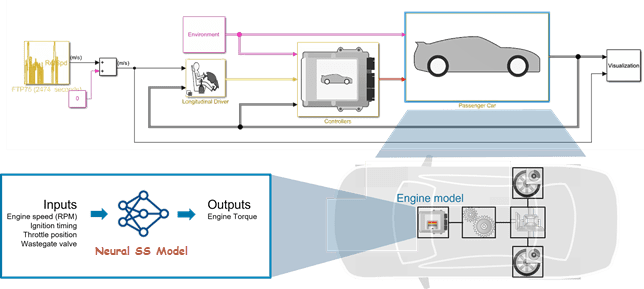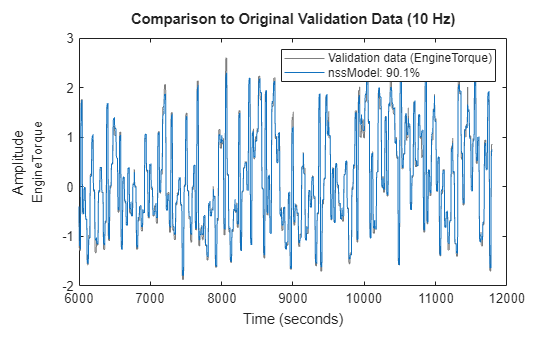# Neural State-Space Model of SI Engine Torque Dynamics

This example describes reduced order modeling (ROM) of the nonlinear torque dynamics of a spark-ignition (SI) engine using a neural state-space model. The identified model can be used for hardware-in-the-loop (HIL) testing, powertrain control, diagnostics, and training algorithm design. For example, you can use the model for aftertreatment control and diagnostic algorithm development. For more information on neural state-space models, see Neural State-Space Models.

You use measurements of the system inputs and outputs to identify the model. This data can come from measurements on a real engine or a high-fidelity model such as one created using the Powertrain Blockset™ SI Reference application.### Data Preparation

Load and display the engine data timetable.

```load SIEngineData IOData stackedplot(IOData) title('SI Engine Signals')```The timetable contains over one hundred thousands observations of five variables measured at 10 Hz.

• Throttle position (degrees)

• Wastegate valve area (aperture percentage)

• Engine speed (RPM)

• Spark timing (degrees)

• Engine torque (N m)

Normalize the data.

`IODataN = normalize(IOData);`

Split the data into estimation (first 60000 data points) and validation (remaining data points) portions.

```eData = IODataN(1:6e4,:); % portion used for estimation vData = IODataN(6e4+1:end,:); % portion used for validation```

Downsample the training data by a factor of 10. This helps speed up the model training process and also limits the focus of the fit to a lower frequency region.

```% Downsample datasets 10 times eDataD = idresamp(eData,[1 10]); vDataD = idresamp(vData,[1 10]); eDataD.Properties.TimeStep```
```ans = duration 1 sec ```
`height(eDataD)`
```ans = 6000 ```

The model training objective is to generate a dynamic system that has the engine torque as output and the other four variables as inputs.

### Neural State-Space Model Identification

Neural state-space models are a type of nonlinear state-space models where the state-transition and measurement functions are modeled using neural networks. In System Identification Toolbox™, they are represented by the `idNeuralStateSpace` object. A nonlinear state-space model has the following form:

`$\begin{array}{l}\stackrel{˙}{\mathit{x}}=\mathit{f}\left(\mathit{x},\mathit{u},\mathit{t},{\theta }_{1}\right)\\ \mathit{y}=\mathit{g}\left(\mathit{x},\mathit{u},\mathit{t},{\theta }_{2}\right)\end{array}$`

Here $x\left(t\right)$ are the state variables, $u\left(t\right)$ are the inputs, and$y\left(t\right)$ are the outputs. Often, there is no explicit dependence on time, so the equations can be reduced to:

`$\begin{array}{l}\stackrel{˙}{\mathit{x}}=\mathit{f}\left(\mathit{x},\mathit{u},{\theta }_{1}\right)\\ \mathit{y}=\mathit{g}\left(\mathit{x},\mathit{u},{\theta }_{2}\right)\end{array}$`

Furthermore, if there are no exogenpous inputs, the equations further simplify to:

`$\begin{array}{l}\stackrel{˙}{\mathit{x}}=\mathit{f}\left(\mathit{x},{\theta }_{1}\right)\\ \mathit{y}=\mathit{g}\left(\mathit{x},{\theta }_{2}\right)\end{array}$`

Here, $\mathit{f}\left(\cdot \right)$ and $\mathit{g}\left(\cdot \right)$ are multi-layer feed-forward networks typically described by `dlnetwork` object of Deep Network Toolbox™. ${\theta }_{1}$ and ${\theta }_{2}$ are identifiable parameters consisting in the weights and biases associated with each layer of these networks. The `nlssest` command can be used to train the $\mathit{f}\left(\cdot \right)$ and $\mathit{g}\left(\cdot \right)$ networks (that is, estimate the values of parameters ${\theta }_{1}$and ${\theta }_{2}$). The training requires that the states of the model be measured directly. Hence a portion of the outputs are the state variables, and the function $\mathit{g}\left(\cdot \right)$ is the identity function for the first ${\mathit{n}}_{\mathit{x}}$ outputs, where ${\mathit{n}}_{\mathit{x}}:=\mathrm{dim}\left(\mathit{x}\right)$.

#### Model Training

Training a neural state-space model requires configuring the model structure by settings the input/output and state dimensions, and the type of the networks $\mathit{f}\left(\cdot \right)$ and $\mathit{g}\left(\cdot \right)$. In this example, you train a one-state (${\mathit{n}}_{\mathit{x}}=1$) model with four outputs. The state variable is the engine torque. It is measured directly and hence is also treated as the model output. Consequently, the model has one output ($\mathit{y}\left(\mathit{t}\right)=\mathit{x}\left(\mathit{t}\right)$).

Begin by designating the input and output signals from the list of variables in the `eData` timetable.

```Inputs = ["ThrottlePosition","WastegateValve","EngineSpeed","SparkTiming"]; Output = "EngineTorque";```

Create a neural state-space model by using the `idNeuralStateSpace` constructor.

```% Define a neural state-space model nx = 1; % number of states = number of outputs nssModel = idNeuralStateSpace(nx,NumInputs=4); nssModel.InputName = Inputs; nssModel.OutputName = Output; % Configure the state network f() nssModel.StateNetwork = createMLPNetwork(nssModel,"state", ... LayerSizes=[128 128], ... WeightsInitializer="glorot", ... BiasInitializer="zeros", ... Activations='tanh')```
```Continuous-time Neural ODE in 1 variables dx/dt = f(x(t),u(t)) y(t) = x(t) + e(t) f(.) network: Deep network with 2 fully connected, hidden layers Activation function: Tanh g(.) network: Deep network with 0 fully connected, hidden layers Activation function: Variables: x1 Status: Created by direct construction or transformation. Not estimated. More information in model's "Report" property. Model Properties ```

Next, prepare the data and the training algorithm options. The data has already been split, downsampled and normalized. You now create multiple data experiments for training by splitting the dataset `eDataD` into overlapping segments. Doing so effectively reduces the prediction horizon from the original data length (6000) to the length of the individual segments. This reduction can sometimes lead to more generalizable results.

```predictionStep = 20; % length of each data segment numExperiment = height(eDataD) - predictionStep; Expts = cell(1, numExperiment); for i = 1:numExperiment Expts{i} = eDataD(i:i+predictionStep,:); if i>1 % set the row time of each segment to be identical; this is a requirement for training a % neural state-space model with multiple data experiments Expts{i}.Properties.RowTimes = Expts{1}.Properties.RowTimes; end end```

Use the `nssTrainingOptions` command to create the set of training options. Pick Adam as the training solver. Set the maximum number of training epochs and the data interpolation method.

```StateOpt = nssTrainingOptions('adam'); StateOpt.MaxEpochs = 90; StateOpt.InputInterSample = 'pchip'; ```

Update the learnable parameters of the neural state-space model `nssModel` by using the `nlssest` command by providing it the training data experiments (`Expts`) and the training options (`StateOpt`). Note that the measurement function $\mathit{g}\left(\mathit{x},\mathit{u},{\theta }_{2}\right)=\mathit{x}\left(\mathit{t}\right)$and does not contain any learnable parameters.

```% Train the neural state-space model tic nssModel = nlssest(Expts,nssModel,StateOpt)```
```Training in progress (completed epoch/max epochs): 0/ 90 1/ 90 2/ 90 3/ 90 4/ 90 5/ 90 6/ 90 7/ 90 8/ 90 9/ 90 10/ 90 11/ 90 12/ 90 13/ 90 14/ 90 15/ 90 16/ 90 17/ 90 18/ 90 19/ 90 20/ 90 21/ 90 22/ 90 23/ 90 24/ 90 25/ 90 26/ 90 27/ 90 28/ 90 29/ 90 30/ 90 31/ 90 32/ 90 33/ 90 34/ 90 35/ 90 36/ 90 37/ 90 38/ 90 39/ 90 40/ 90 41/ 90 42/ 90 43/ 90 44/ 90 45/ 90 46/ 90 47/ 90 48/ 90 49/ 90 50/ 90 51/ 90 52/ 90 53/ 90 54/ 90 55/ 90 56/ 90 57/ 90 58/ 90 59/ 90 60/ 90 61/ 90 62/ 90 63/ 90 64/ 90 65/ 90 66/ 90 67/ 90 68/ 90 69/ 90 70/ 90 71/ 90 72/ 90 73/ 90 74/ 90 75/ 90 76/ 90 77/ 90 78/ 90 79/ 90 80/ 90 81/ 90 82/ 90 83/ 90 84/ 90 85/ 90 86/ 90 87/ 90 88/ 90 89/ 90 `````` 90/ 90 Generating estimation report...done. Continuous-time Neural ODE in 1 variables dx/dt = f(x(t),u(t)) y(t) = x(t) + e(t) f(.) network: Deep network with 2 fully connected, hidden layers Activation function: Tanh g(.) network: Deep network with 0 fully connected, hidden layers Activation function: Variables: x1 Status: Estimated using NLSSEST on time domain data "Expts". Fit to estimation data: [-44.21 -49.85 -57.25 -67.86 -83.07 -105.3 -142.1 -272.2 -313.2 -27.87....% FPE: 0.01088, MSE: [0.008885 0.009086 0.009231 0.009365 0.009495 0.009436 0.009204 0.009095 0.009087 0.00869... More information in model's "Report" property. Model Properties ```
`toc`
```Elapsed time is 2185.697275 seconds. ```

### Result Validation

Run the compare command to compare the simulated response of the model agains the estimation dataset (`eDataD`) and the validation dataset (`vDataD`). Note that while the model was trained on segments 20 samples long (effectively reducing the prediction horizon to 20), it is validated by running a full simulation (horizon of ~6000).

```clf subplot(211) compare(eDataD,nssModel) % compare against estimation data title('Neural State-Space Model: Comparison to Estimation Data') subplot(212) compare(vDataD,nssModel) % compare against validation data title('Comparison to Validation Data (Downsampled)')```Note that the training and validation has been performed on the downsampled datasets. You can also validate the quality on the dataset with the original sample time of 0.1 seconds. This can be done by using the compare command again, as follows:

```clf compare(vData,nssModel) % compare against original validation data (sample rate of 10 Hz) title('Comparison to Original Validation Data (10 Hz)')```The comparison is possible because the model `nssModel` is a continuous-time model, which can be compared against a reference dataset of any sampling rate. The validation plots show the good quality of the identified model.

You can also verify the model quality by pure simulation using the `sim` command in MATLAB® or by putting it in a Simulink® model using the dedicated `Neural` `SS` `Model` block.

```% Create an iddata representation of the data in vData to feed the signals to the Simulink % model using an IDDATA Source block. zsim = iddata(vData,InputName=Inputs,OutputName=Output); zsim.Tstart = 0; % Open the simulation model and simulate it using zsim as the source of input data. mdl = 'nlss_simulator'; tstop = zsim.SamplingInstants(end); Ts = zsim.Ts; open_system(mdl)````sim(mdl);`